# 模型可视化¶

## 概述¶

VisualDL 是一个面向深度学习任务设计的可视化工具。VisualDL 利用了丰富的图表来展示数据，用户可以更直观、清晰地查看数据的特征与变化趋势，有助于分析数据、及时发现错误，进而改进神经网络模型的设计。

Scalar 折线图 动态展示损失函数值、准确率等标量数据
Image 图片可视化 显示图片，可显示输入图片和处理后的结果，便于查看中间过程的变化
Audio 音频播放 播放训练过程中的音频数据，监控语音识别与合成等任务的训练过程
Graph 网络结构 展示网络结构、节点属性及数据流向，辅助学习、优化网络结构
Histogram 直方图 展示训练过程中权重、梯度等张量的分布
PR Curve 折线图 权衡精度与召回率之间的平衡关系，便于选择最佳阈值
High Dimensional 数据降维 将高维数据映射到 2D/3D 空间来可视化嵌入，便于观察不同数据的相关性

## Scalar -- 折线图组件¶

### 介绍¶

Scalar 组件的输入数据类型为标量，该组件的作用是将训练参数以折线图形式呈现。将损失函数值、准确率等标量数据作为参数传入 scalar 组件，即可画出折线图，便于观察变化趋势。

### 记录接口¶

Scalar 组件的记录接口如下：

```add_scalar(tag, value, step, walltime=None)
```

tag string 记录指标的标志，如`train/loss`，不能含有`%`
value float 要记录的数据值
step int 记录的步数
walltime int 记录数据的时间戳，默认为当前时间戳

### Demo¶

• 基础使用

```from visualdl import LogWriter

if __name__ == '__main__':
value = [i/1000.0 for i in range(1000)]
# 初始化一个记录器
with LogWriter(logdir="./log/scalar_test/train") as writer:
for step in range(1000):
# 向记录器添加一个tag为`acc`的数据
# 向记录器添加一个tag为`loss`的数据
writer.add_scalar(tag="loss", step=step, value=1/(value[step] + 1))
```

```visualdl --logdir ./log --port 8080
```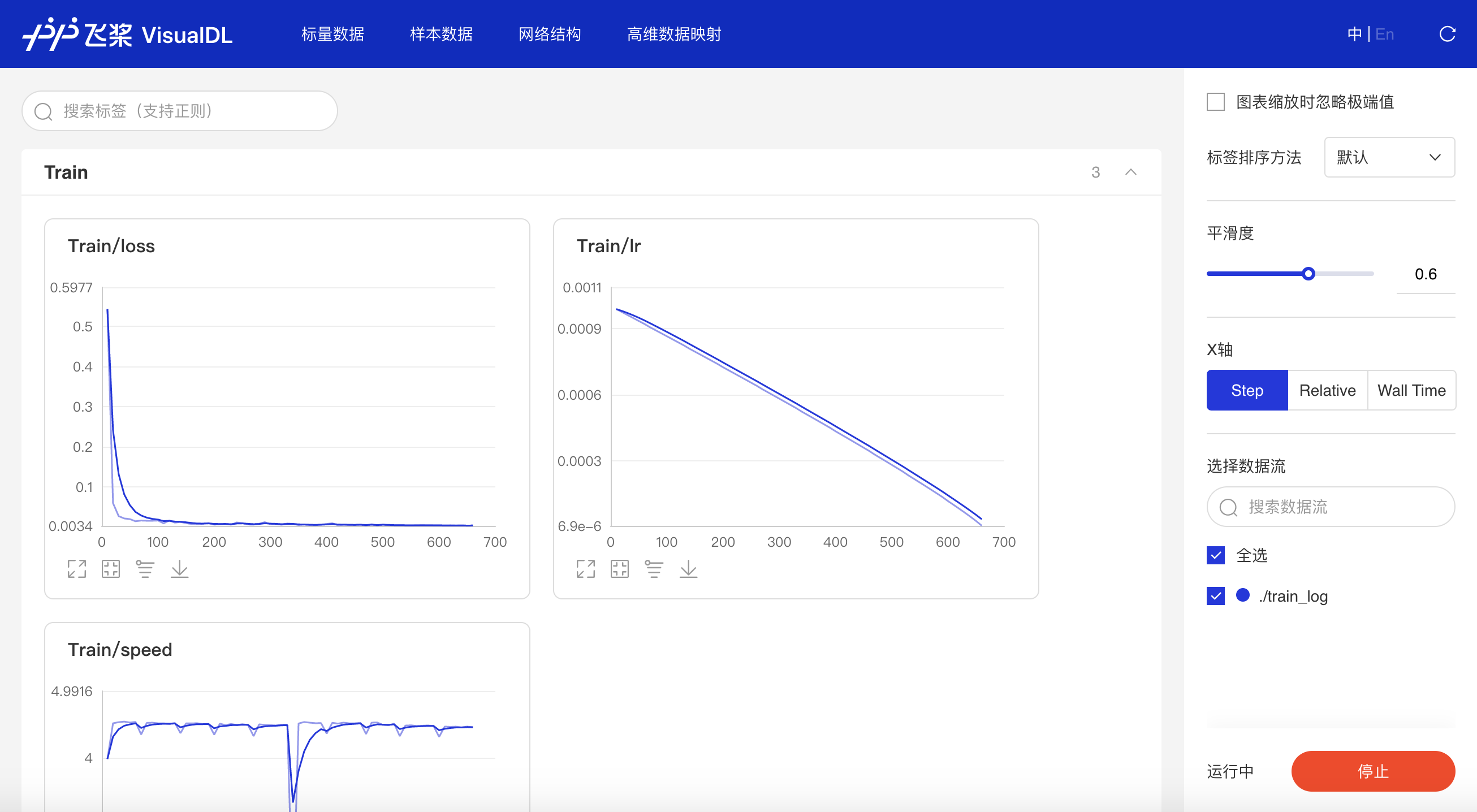• 多组实验对比

1. 创建子日志文件储存每组实验的参数数据

2. 将数据写入scalar组件时，使用相同的tag，即可实现对比不同实验同一类型参数

```from visualdl import LogWriter

if __name__ == '__main__':
value = [i/1000.0 for i in range(1000)]
# 步骤一：创建父文件夹：log与子文件夹：scalar_test
with LogWriter(logdir="./log/scalar_test") as writer:
for step in range(1000):
# 步骤二：向记录器添加一个tag为`train/acc`的数据
# 步骤二：向记录器添加一个tag为`train/loss`的数据
writer.add_scalar(tag="train/loss", step=step, value=1/(value[step] + 1))
# 步骤一：创建第二个子文件夹scalar_test2
value = [i/500.0 for i in range(1000)]
with LogWriter(logdir="./log/scalar_test2") as writer:
for step in range(1000):
# 步骤二：在同样名为`train/acc`下添加scalar_test2的accuracy的数据
# 步骤二：在同样名为`train/loss`下添加scalar_test2的loss的数据
writer.add_scalar(tag="train/loss", step=step, value=1/(value[step] + 1))
```

```visualdl --logdir ./log --port 8080
```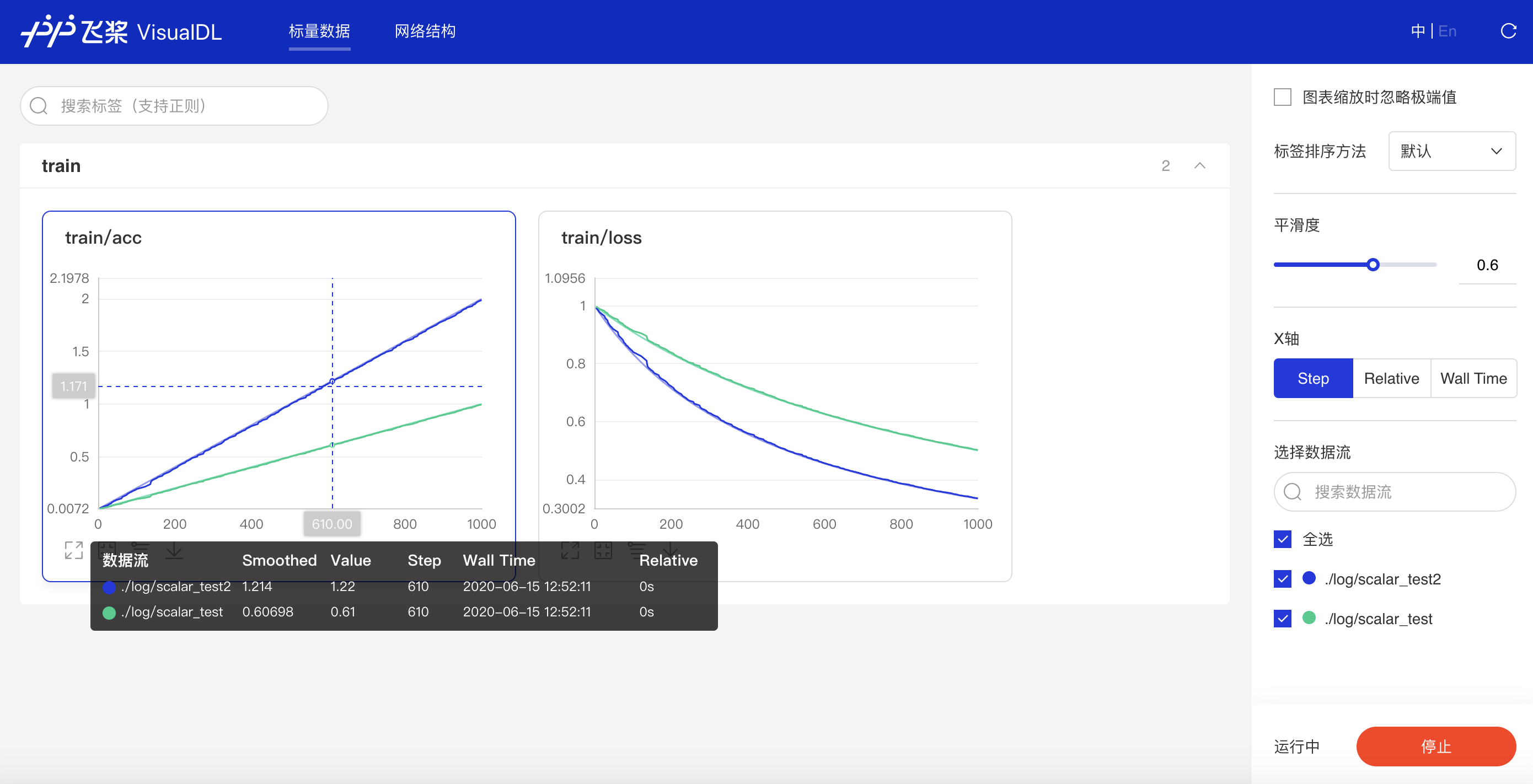*多组实验对比的应用案例可参考AI Studio项目：VisualDL 2.0--眼疾识别训练可视化

### 功能操作说明¶

• 支持数据卡片「最大化」、「还原」、「坐标系转化」（y轴对数坐标）、「下载」折线图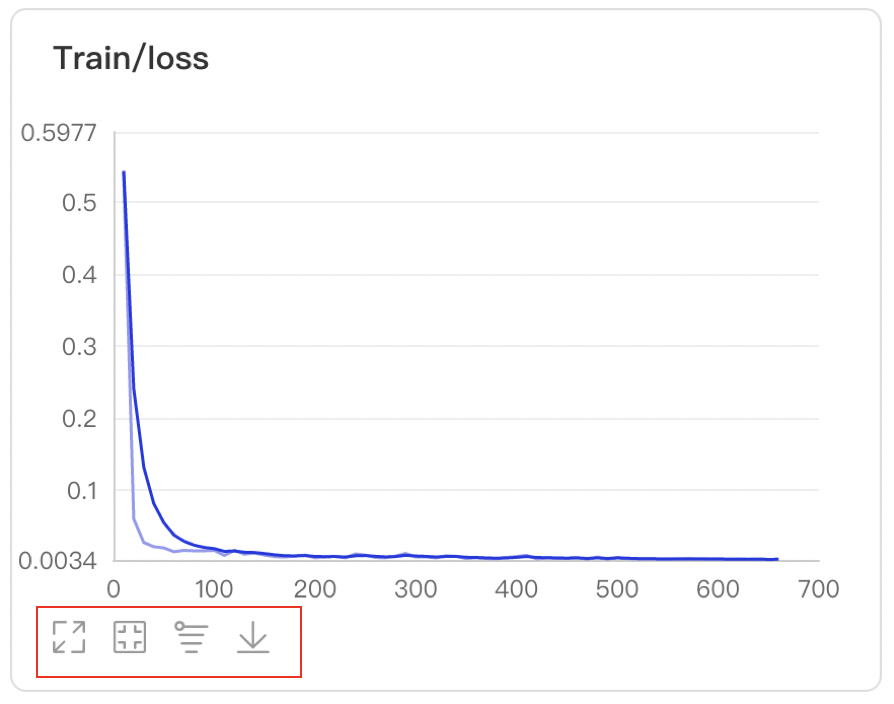• 数据点Hover展示详细信息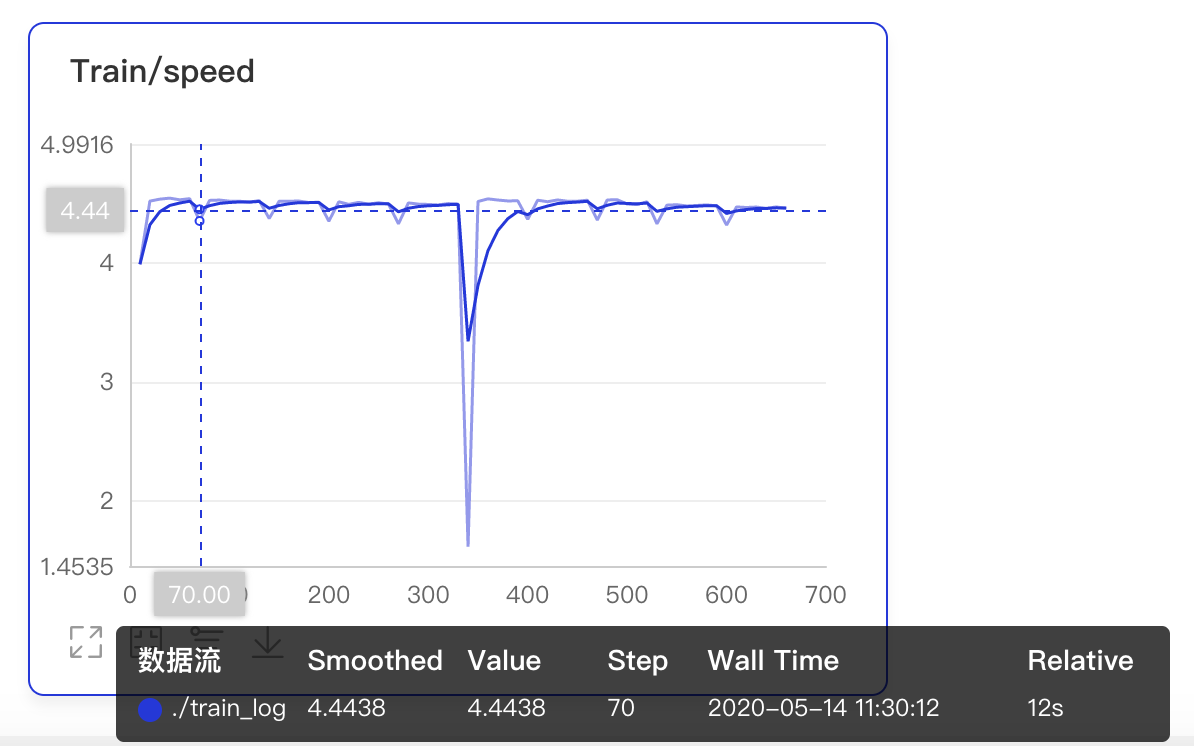• 可搜索卡片标签，展示目标图像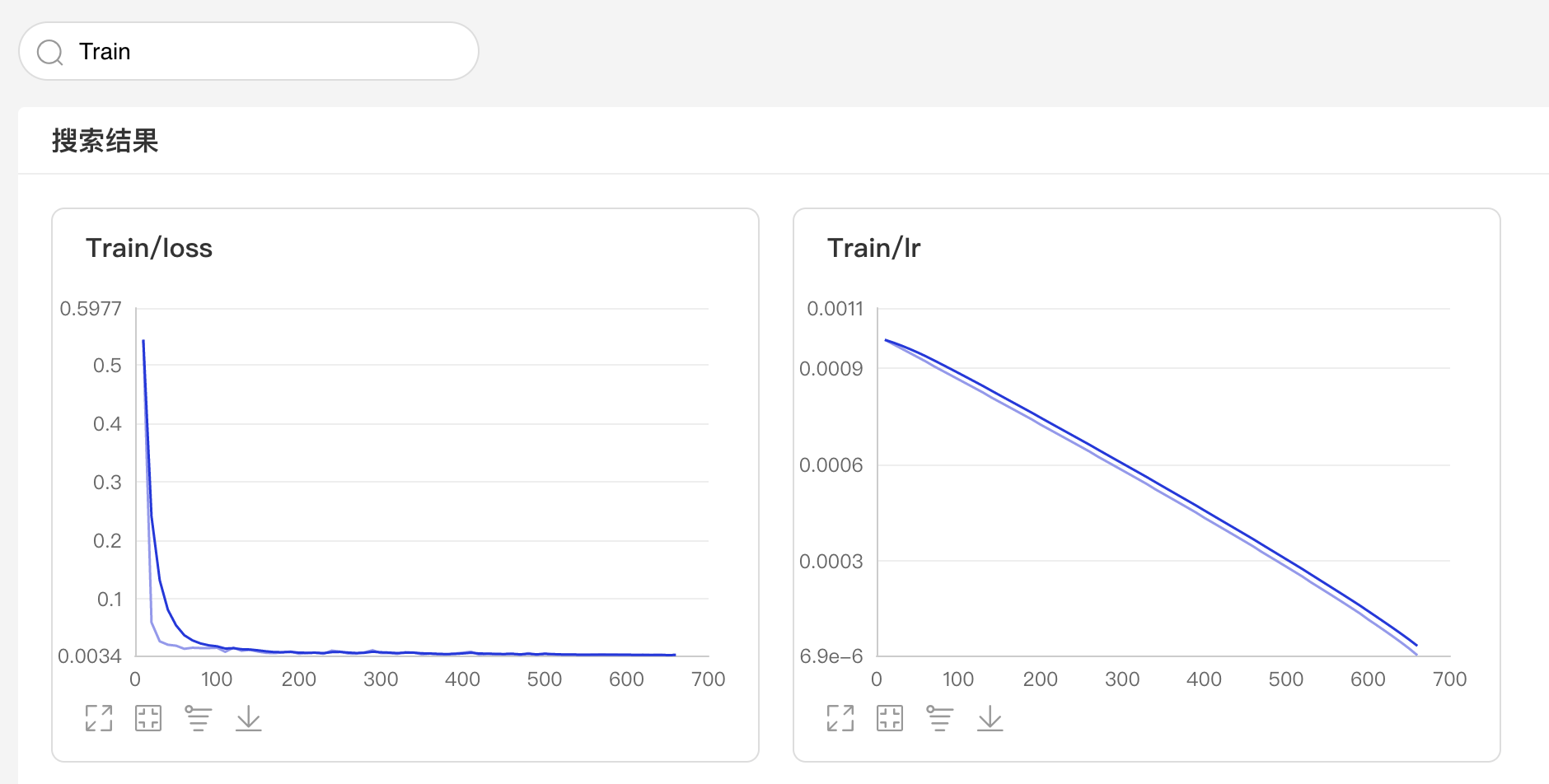• 可搜索打点数据标签，展示特定数据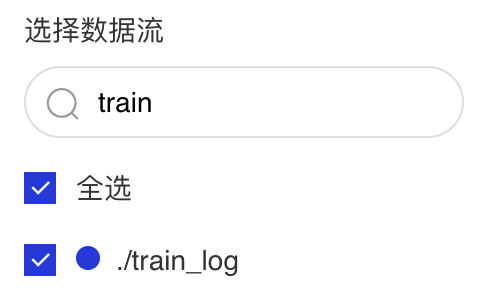• X轴有三种衡量尺度

1. Step：迭代次数

2. Walltime：训练绝对时间

3. Relative：训练时长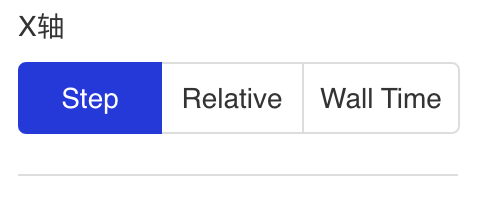• 可调整曲线平滑度，以便更好的展现参数整体的变化趋势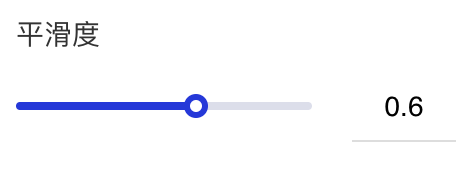## Image -- 图片可视化组件¶

### 介绍¶

Image 组件用于显示图片数据随训练的变化。在模型训练过程中，将图片数据传入 Image 组件，就可在 VisualDL 的前端网页查看相应图片。

### 记录接口¶

Image 组件的记录接口如下：

```add_image(tag, img, step, walltime=None)
```

tag string 记录指标的标志，如`train/loss`，不能含有`%`
img numpy.ndarray 以ndarray格式表示的图片
step int 记录的步数
walltime int 记录数据的时间戳，默认为当前时间戳

### Demo¶

```import numpy as np
from PIL import Image
from visualdl import LogWriter

def random_crop(img):
"""获取图片的随机 100x100 分片
"""
img = Image.open(img)
w, h = img.size
random_w = np.random.randint(0, w - 100)
random_h = np.random.randint(0, h - 100)
r = img.crop((random_w, random_h, random_w + 100, random_h + 100))
return np.asarray(r)

if __name__ == '__main__':
# 初始化一个记录器
with LogWriter(logdir="./log/image_test/train") as writer:
for step in range(6):
# 添加一个图片数据
img=random_crop("../../docs/images/eye.jpg"),
step=step)
```

```visualdl --logdir ./log --port 8080
```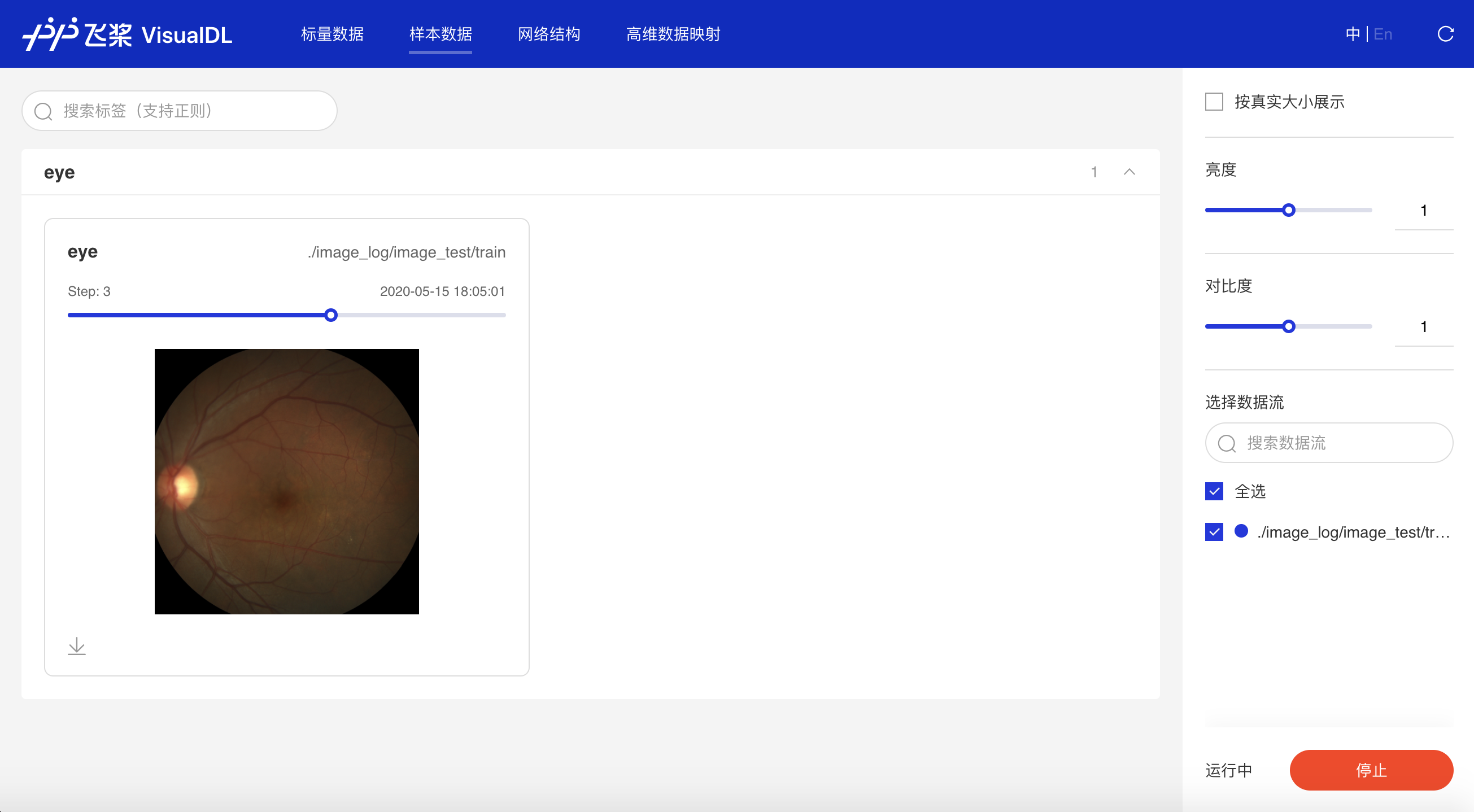### 功能操作说明¶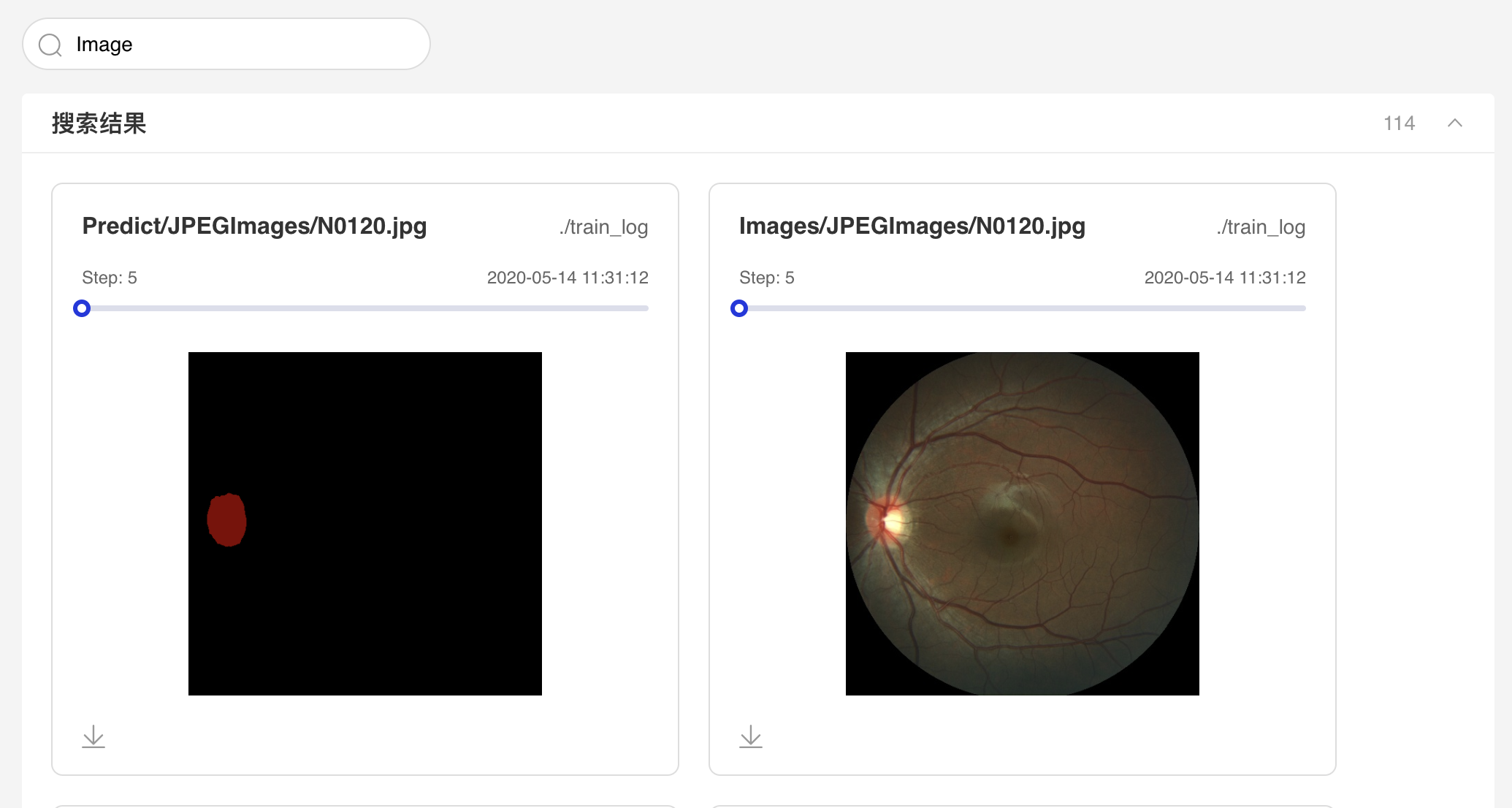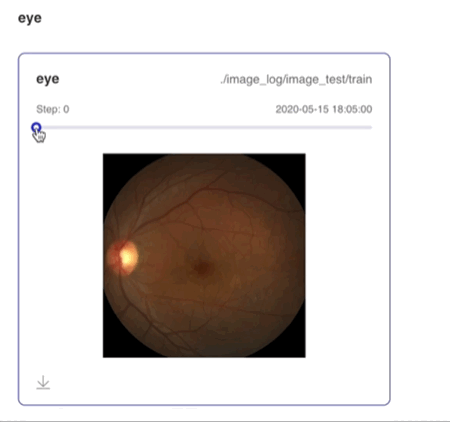## Audio--音频播放组件¶

### 介绍¶

Audio组件实时查看训练过程中的音频数据，监控语音识别与合成等任务的训练过程。

### 记录接口¶

Audio 组件的记录接口如下：

```add_audio(tag, audio_array, step, sample_rate)
```

tag string 记录指标的标志，如`audio_tag`，不能含有`%`
audio_arry numpy.ndarray 以ndarray格式表示的音频
step int 记录的步数
sample_rate int 采样率，注意正确填写对应音频的原采样率

### Demo¶

```from visualdl import LogWriter
import numpy as np
import wave

"""
Get audio data.
"""
CHUNK = 4096
f = wave.open(audio_path, "rb")
wavdata = []
while chunk:
data = np.frombuffer(chunk, dtype='uint8')
wavdata.extend(data)
# 8k sample rate, 16bit frame, 1 channel
shape = [8000, 2, 1]
return shape, wavdata

if __name__ == '__main__':
with LogWriter(logdir="./log") as writer:
audio_shape, audio_data = read_audio_data("./testing.wav")
audio_data = np.array(audio_data)
audio_array=audio_data,
step=0,
sample_rate=8000)
```

```visualdl --logdir ./log --port 8080
```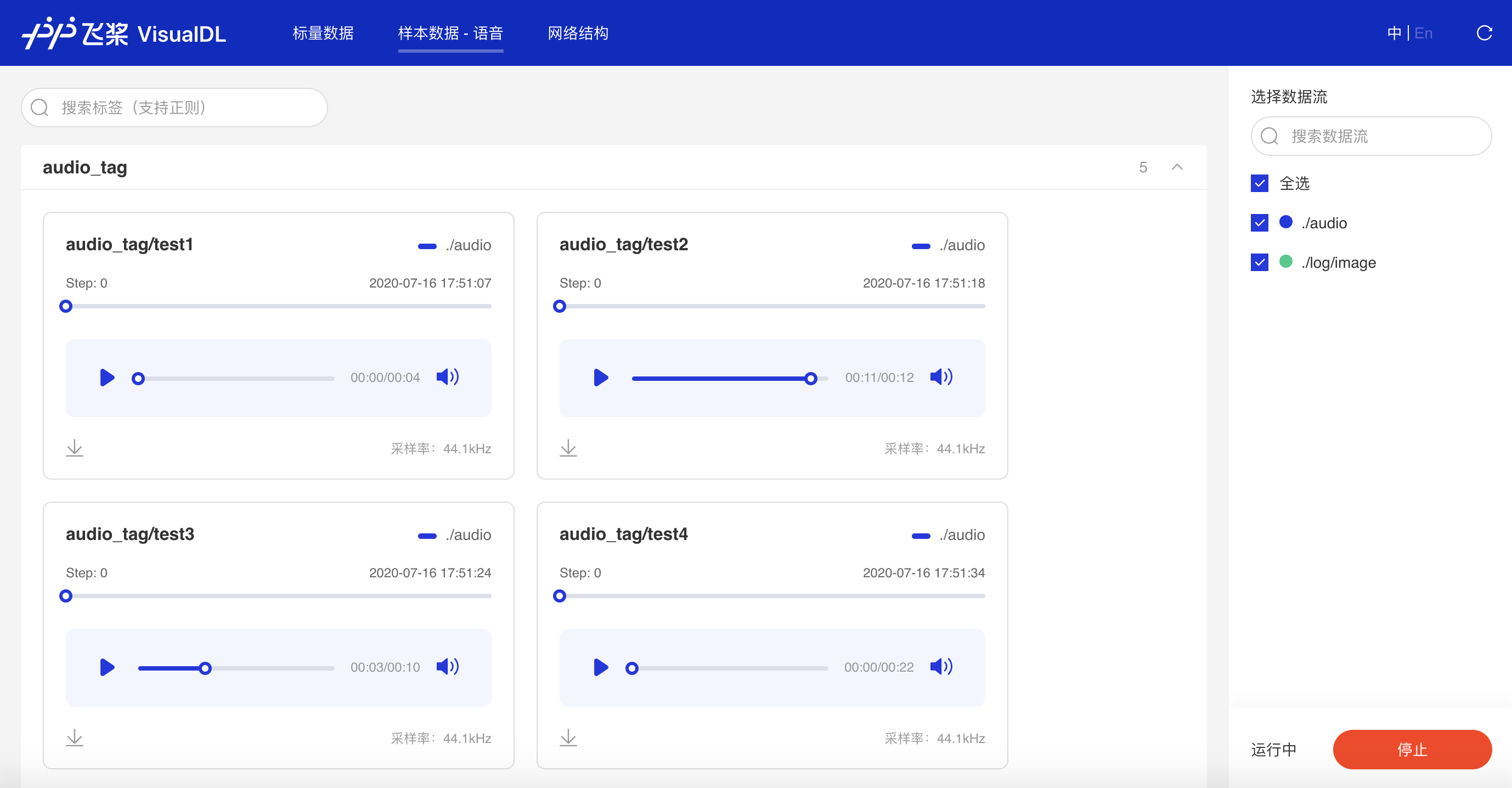### 功能操作说明¶

• 可搜索音频标签显示对应音频数据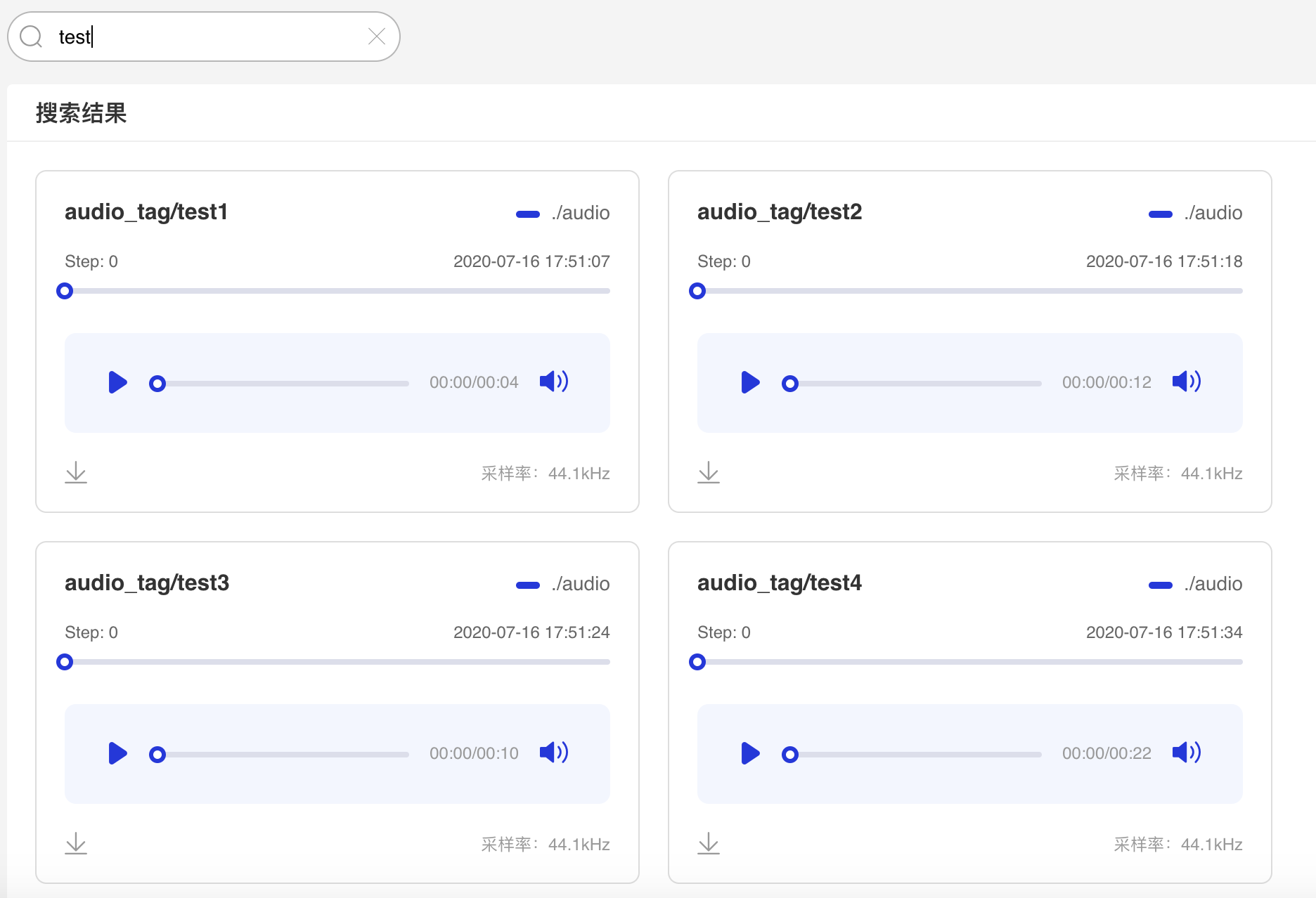• 支持滑动Step/迭代次数试听不同迭代次数下的音频数据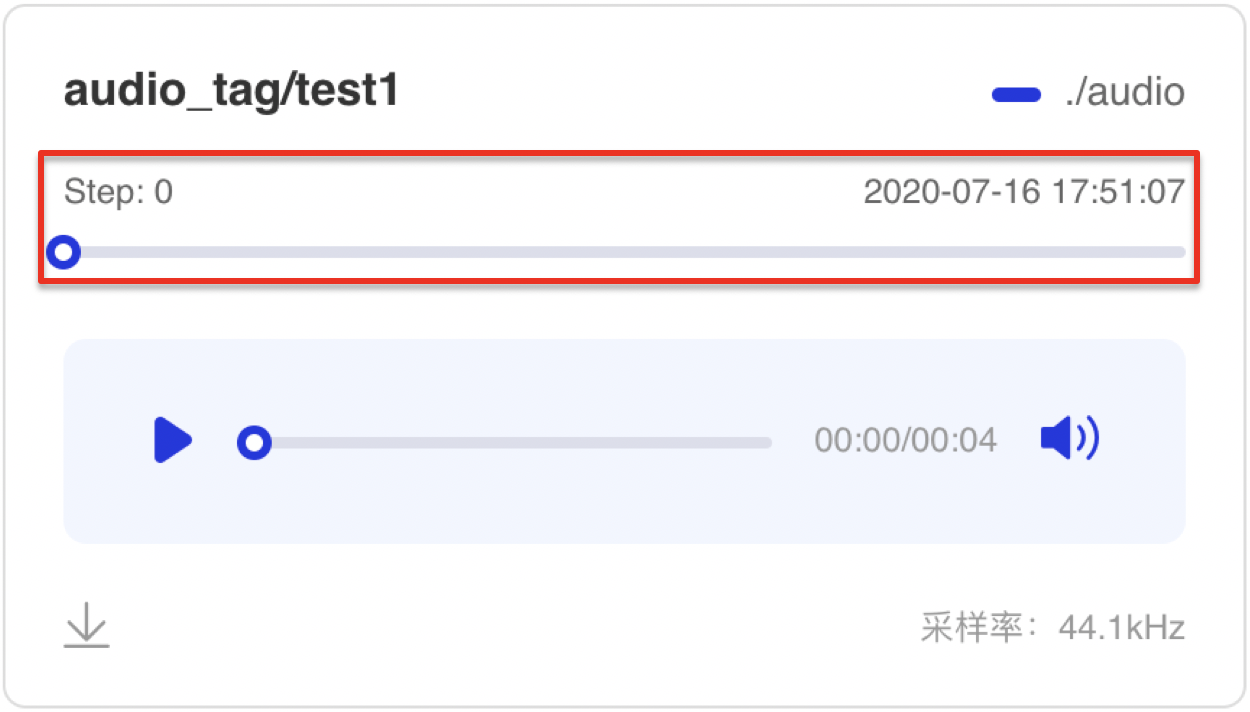• 支持播放/暂停音频数据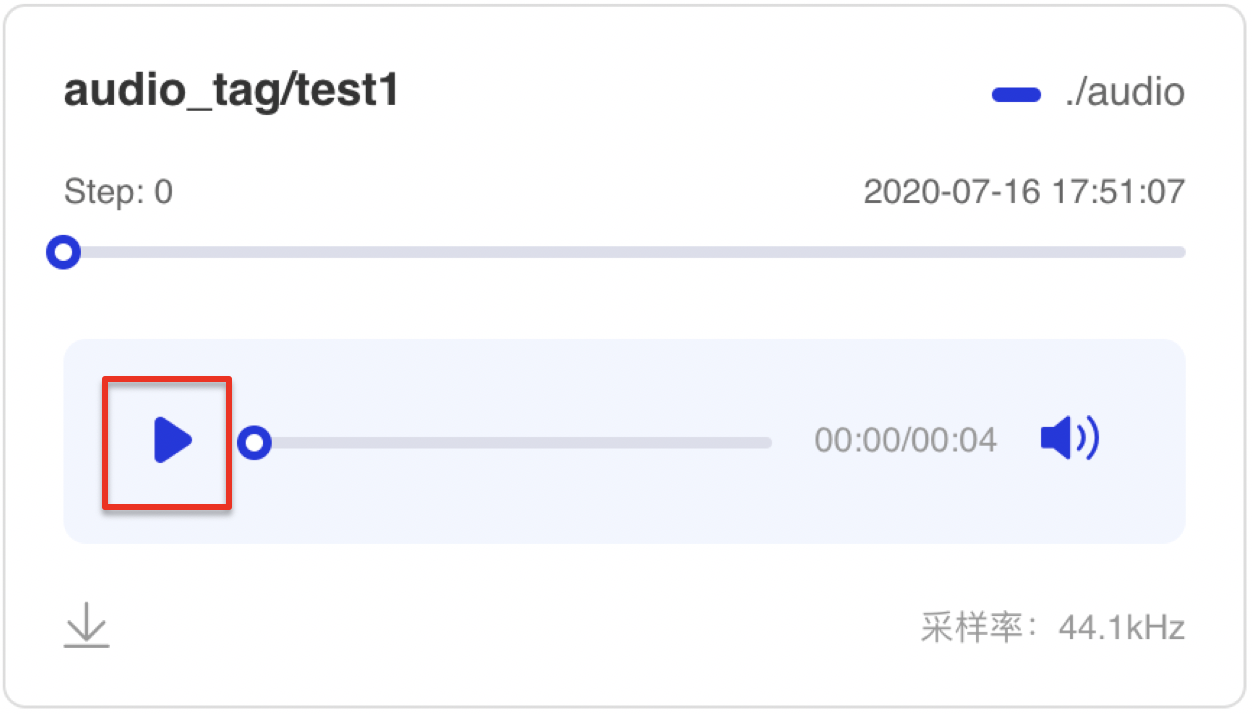• 支持音量调节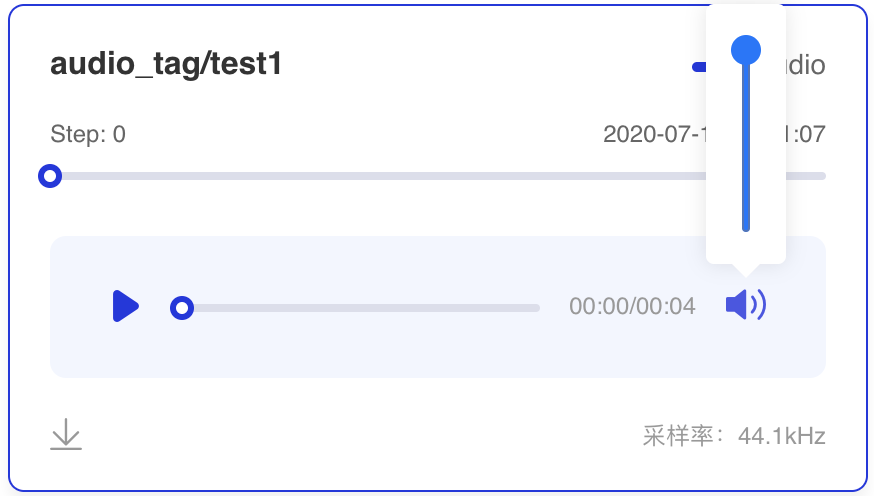• 支持音频下载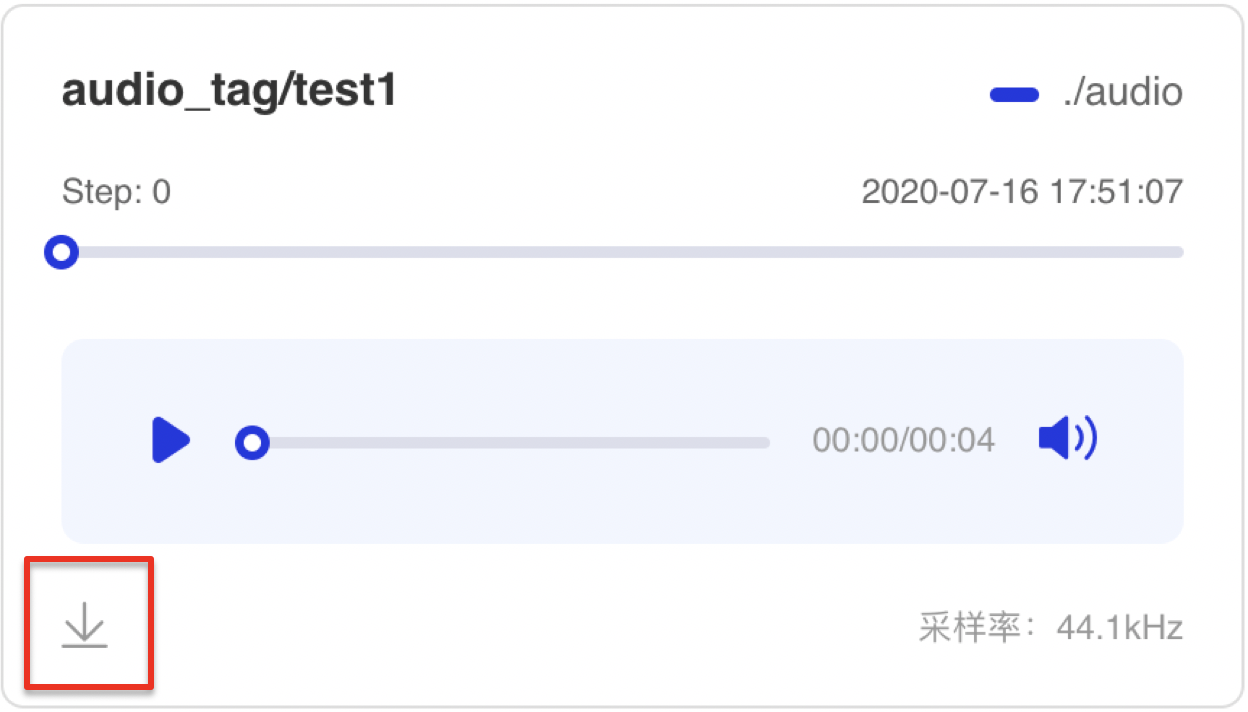## Graph--网络结构组件¶

### 介绍¶

Graph组件一键可视化模型的网络结构。用于查看模型属性、节点信息、节点输入输出等，并进行节点搜索，协助开发者们快速分析模型结构与了解数据流向。

### Demo¶

• 前端模型文件拖拽上传：

• 如只需使用Graph组件，则无需添加任何参数，在命令行执行`visualdl`后即可启动面板进行上传。

• 如果同时需使用其他功能，在命令行指定日志文件路径（以`./log`为例）即可启动面板进行上传：

```visualdl --logdir ./log --port 8080
```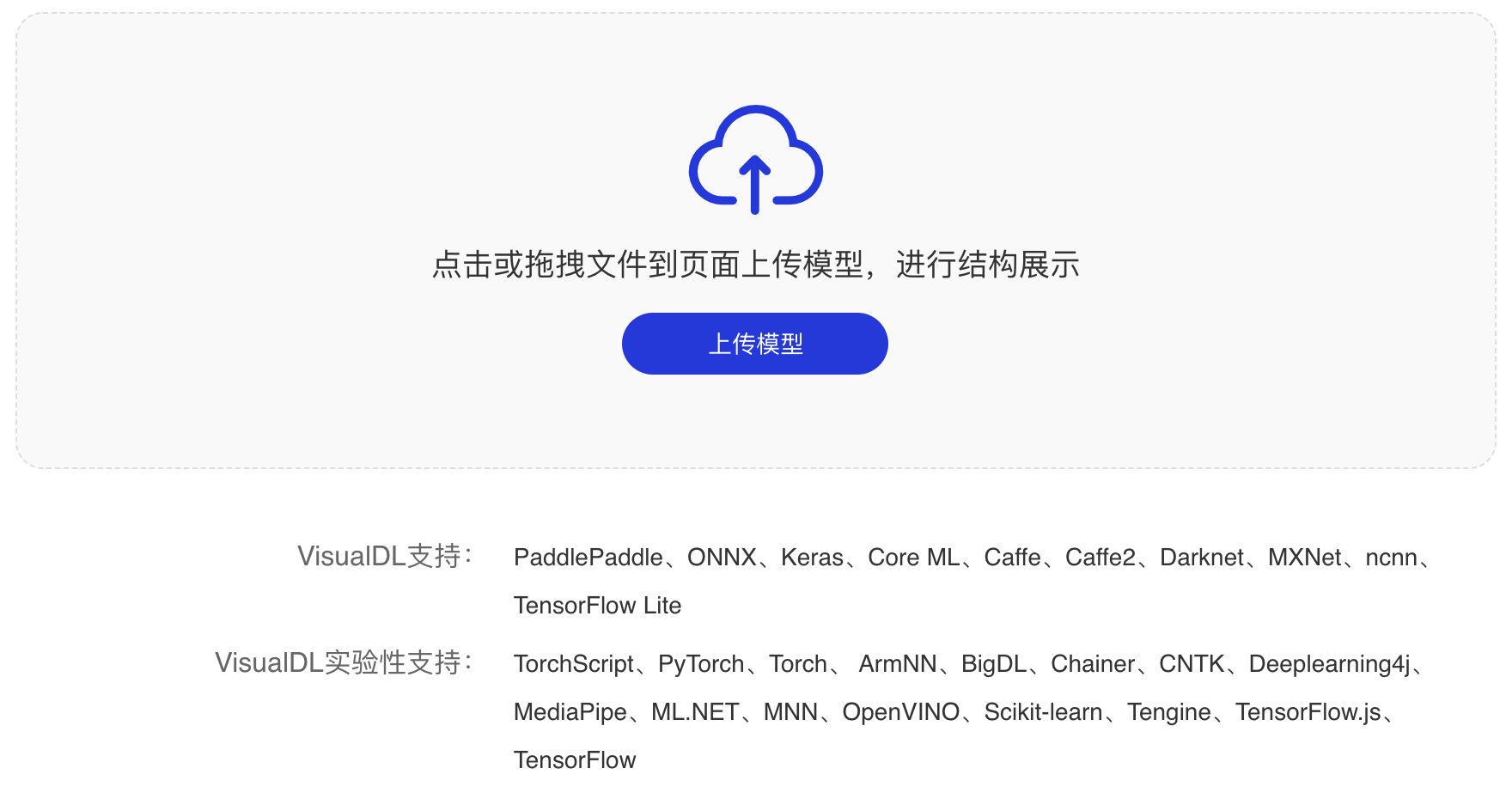• 后端启动Graph：

• 在命令行加入参数`--model`并指定模型文件路径（非文件夹路径），即可启动并查看网络结构可视化：

```visualdl --model ./log/model --port 8080
```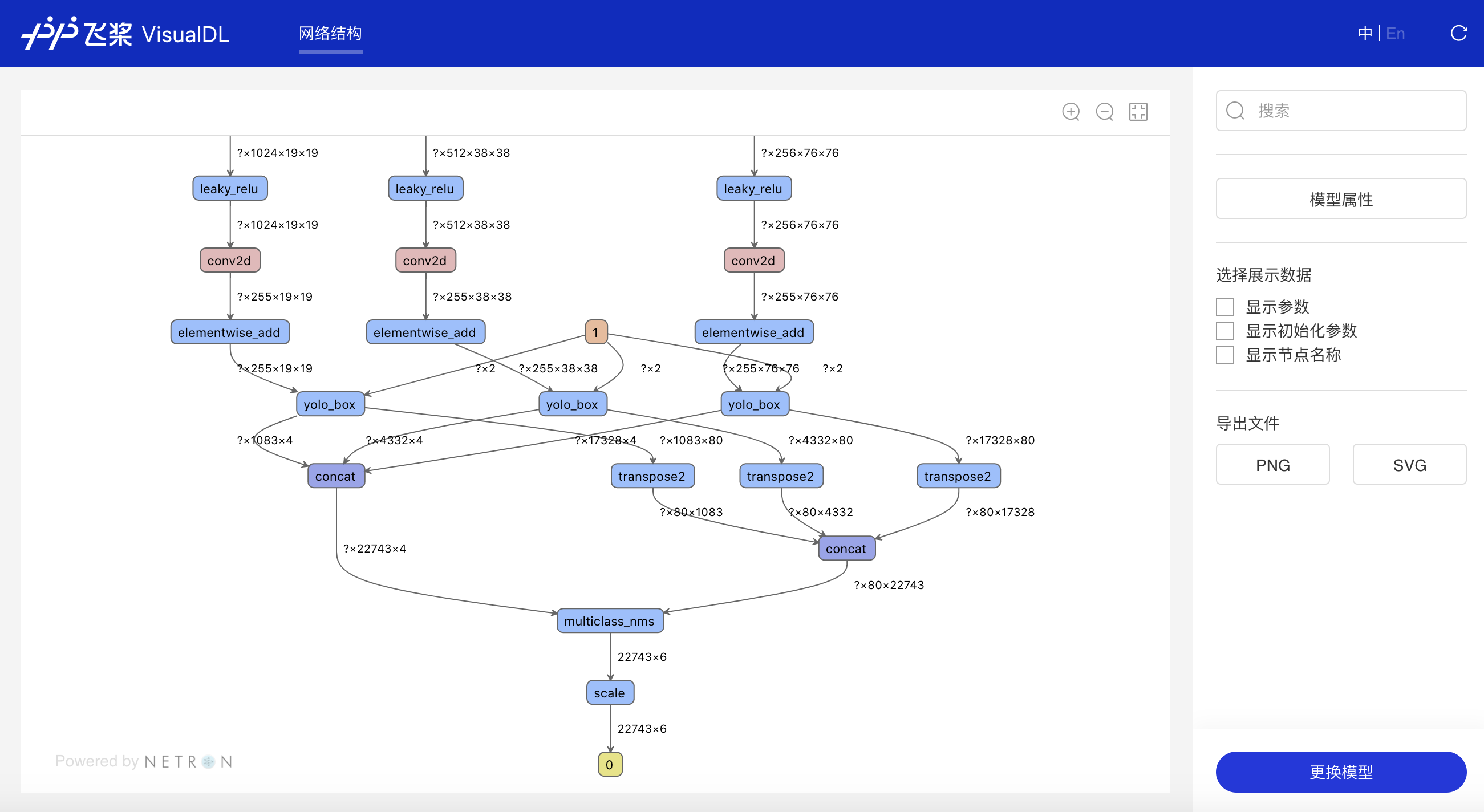### 功能操作说明¶

• 一键上传模型

• 实验性支持模型格式：TorchScript、PyTorch、Torch、 ArmNN、BigDL、Chainer、CNTK、Deeplearning4j、MediaPipe、ML.NET、MNN、OpenVINO、Scikit-learn、Tengine、TensorFlow.js、TensorFlow• 支持上下左右任意拖拽模型、放大和缩小模型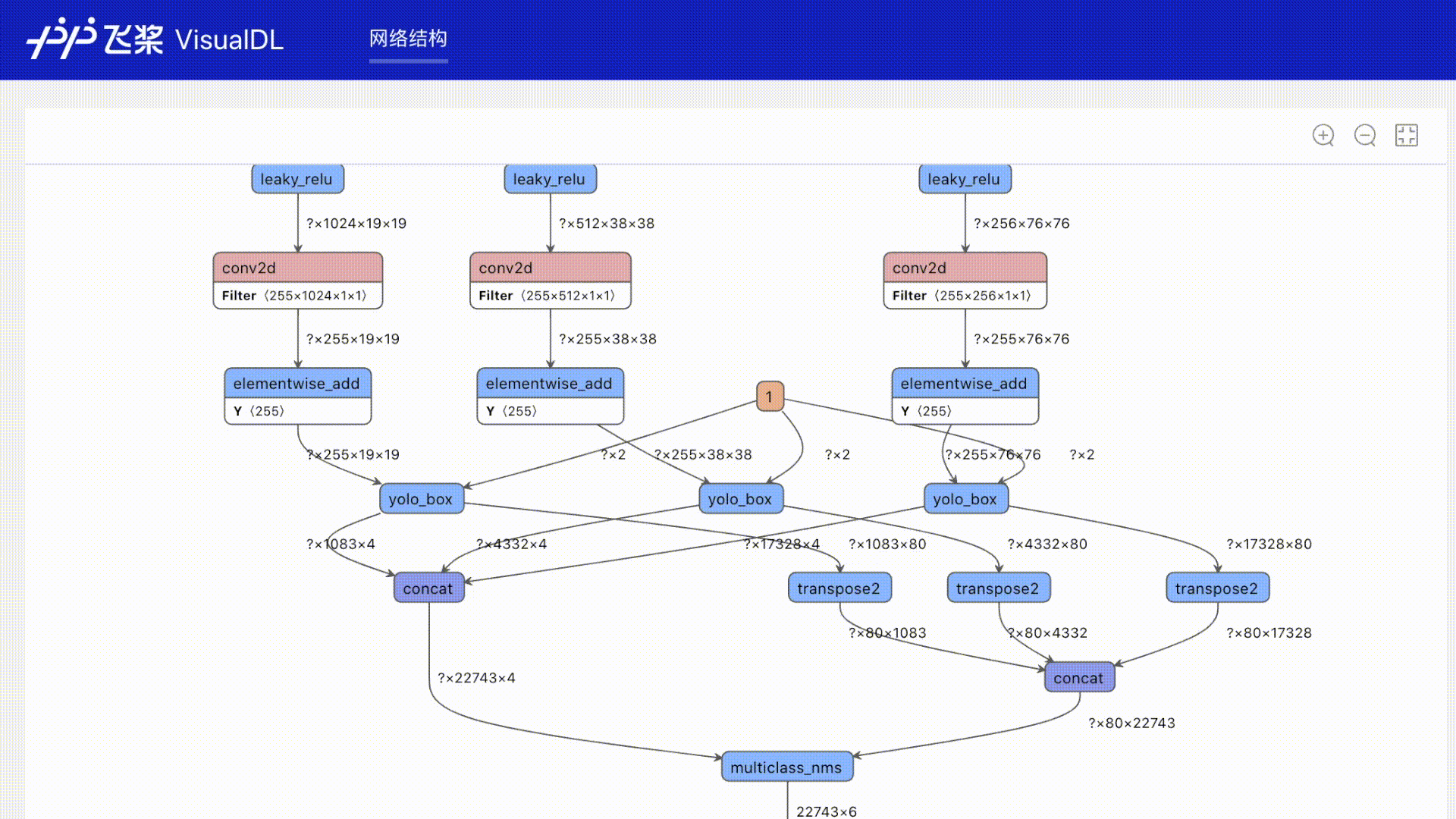• 搜索定位到对应节点• 点击查看模型属性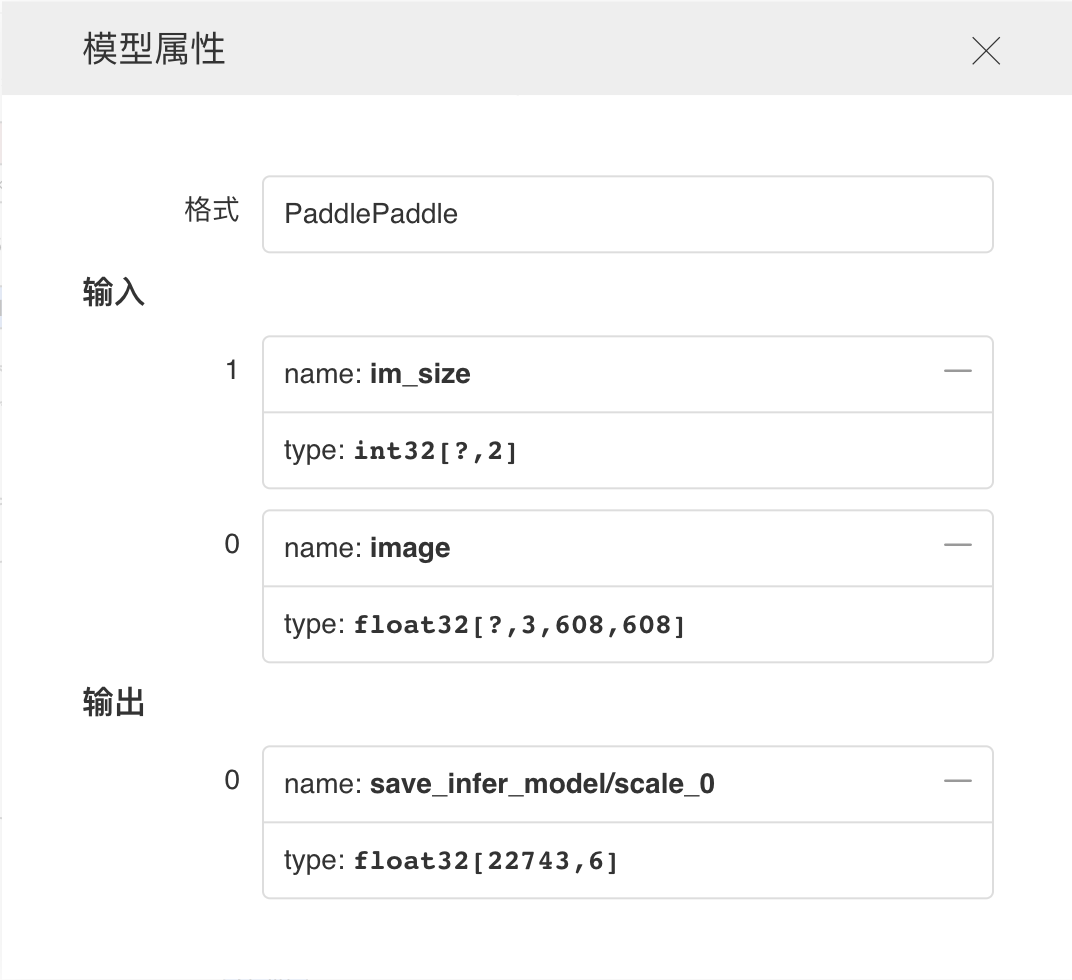• 支持选择模型展示的信息• 支持以PNG、SVG格式导出模型结构图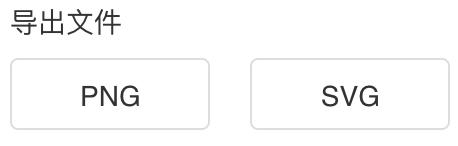• 点击节点即可展示对应属性信息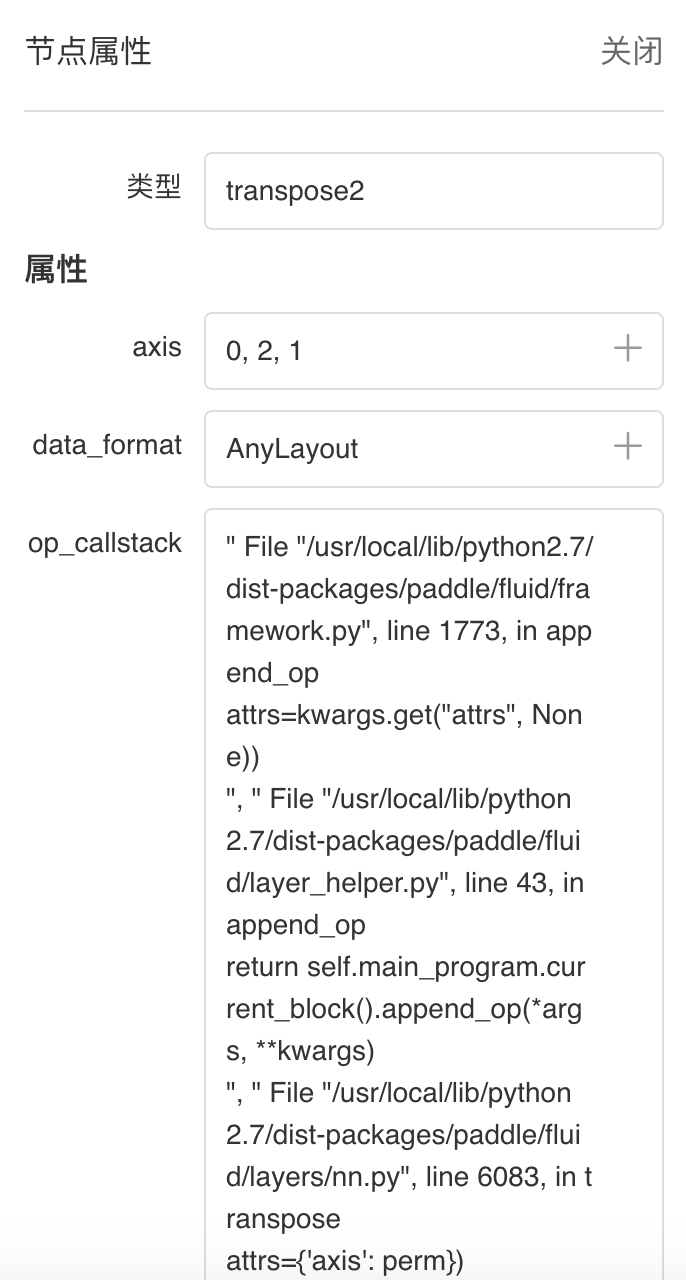• 支持一键更换模型## Histogram--直方图组件¶

### 记录接口¶

Histogram 组件的记录接口如下：

```add_histogram(tag, values, step, walltime=None, buckets=10)
```

tag string 记录指标的标志，如`train/loss`，不能含有`%`
values numpy.ndarray or list 以ndarray或list格式表示的数据
step int 记录的步数
walltime int 记录数据的时间戳，默认为当前时间戳
buckets int 生成直方图的分段数，默认为10

### Demo¶

```from visualdl import LogWriter
import numpy as np

if __name__ == '__main__':
values = np.arange(0, 1000)
with LogWriter(logdir="./log/histogram_test/train") as writer:
for index in range(1, 101):
interval_start = 1 + 2 * index / 100.0
interval_end = 6 - 2 * index / 100.0
data = np.random.uniform(interval_start, interval_end, size=(10000))
values=data,
step=index,
buckets=10)
```

```visualdl --logdir ./log --port 8080
```

### 功能操作说明¶

• 支持数据卡片「最大化」、直方图「下载」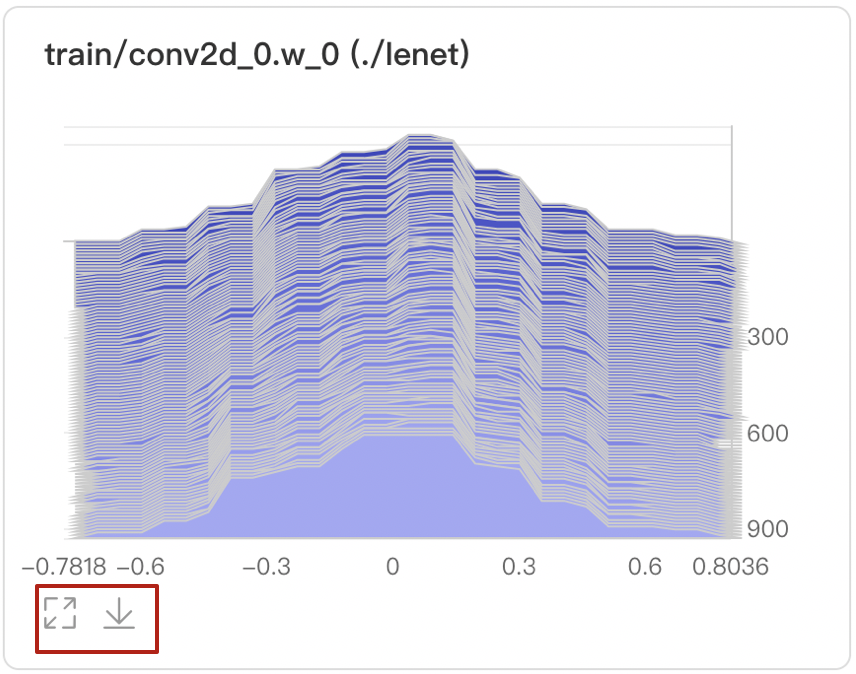• 可选择Offset或Overlay模式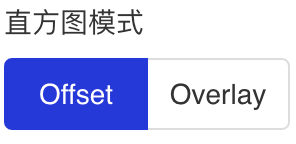• Offset模式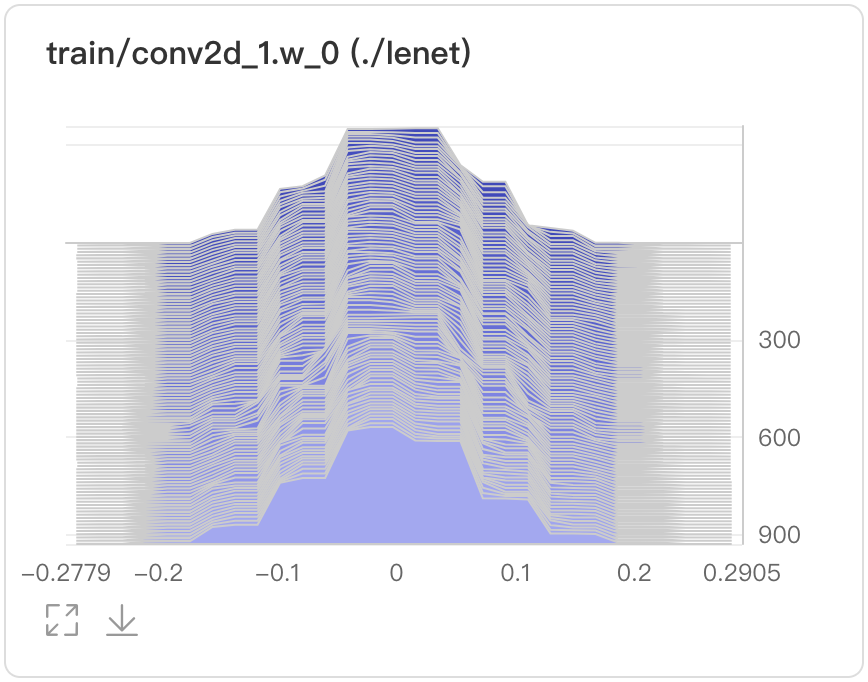• Overlay模式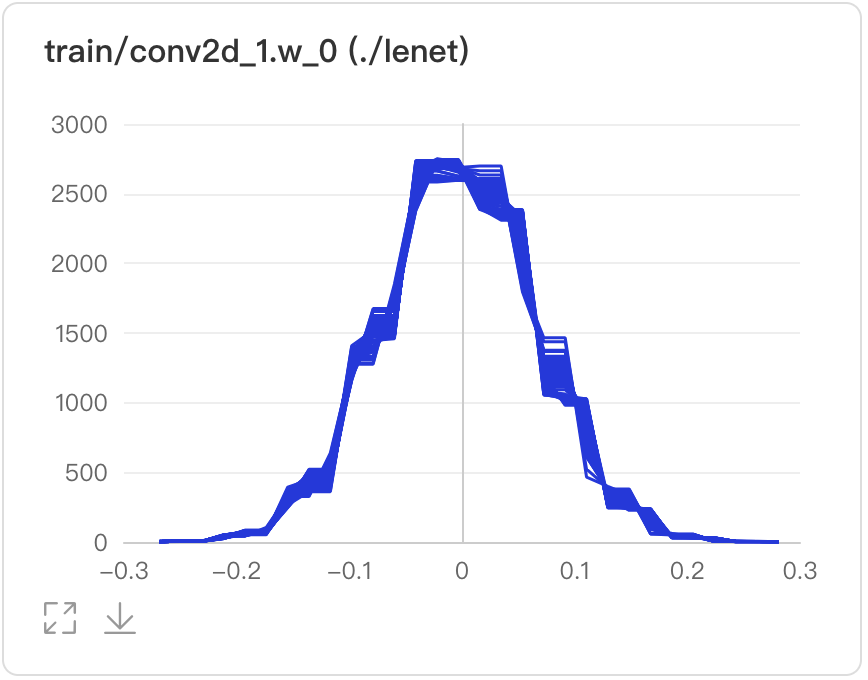• 数据点Hover展示参数值、训练步数、频次

• 在第240次训练步数时，权重为-0.0031，且出现的频次是2734次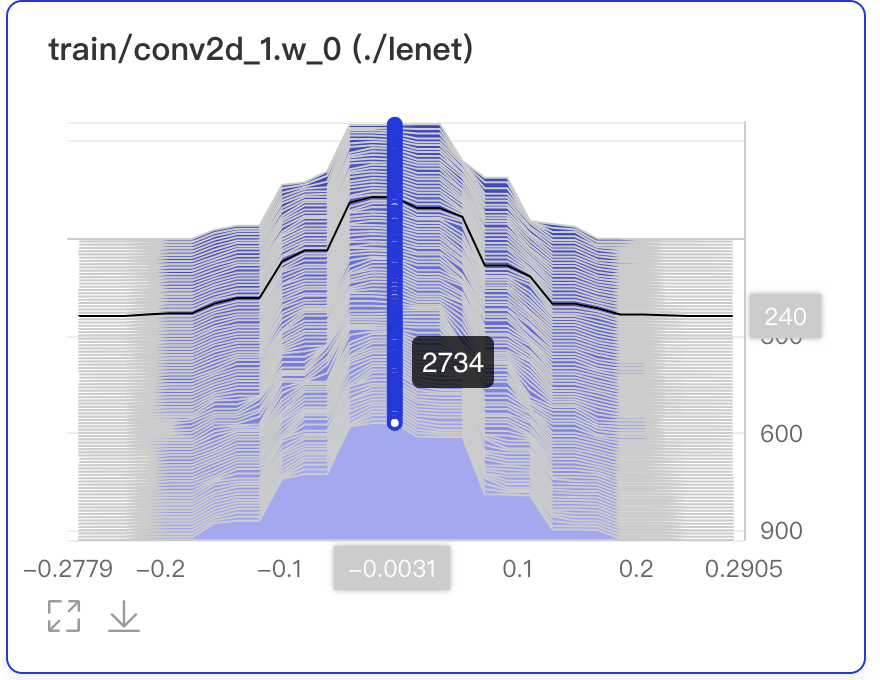• 可搜索卡片标签，展示目标直方图• 可搜索打点数据标签，展示特定数据流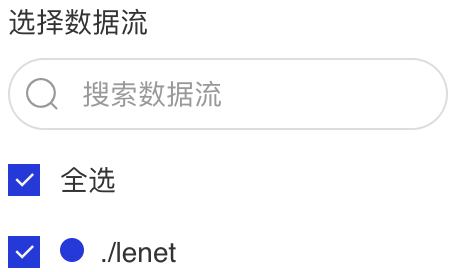## PR Curve--PR曲线组件¶

### 介绍¶

PR Curve以折线图形式呈现精度与召回率的权衡分析，清晰直观了解模型训练效果，便于分析模型是否达到理想标准。

### 记录接口¶

PR Curve组件的记录接口如下：

```add_pr_curve(tag, labels, predictions, step=None, num_thresholds=10)
```

tag string 记录指标的标志，如`train/loss`，不能含有`%`
labels numpy.ndarray or list 以ndarray或list格式表示的实际类别
predictions numpy.ndarray or list 以ndarray或list格式表示的预测类别
step int 记录的步数
num_thresholds int 阈值设置的个数，默认为10，最大值为127

### Demo¶

```from visualdl import LogWriter
import numpy as np

with LogWriter("./log/pr_curve_test/train") as writer:
for step in range(3):
labels = np.random.randint(2, size=100)
predictions = np.random.rand(100)
labels=labels,
predictions=predictions,
step=step,
num_thresholds=5)
```

```visualdl --logdir ./log --port 8080
```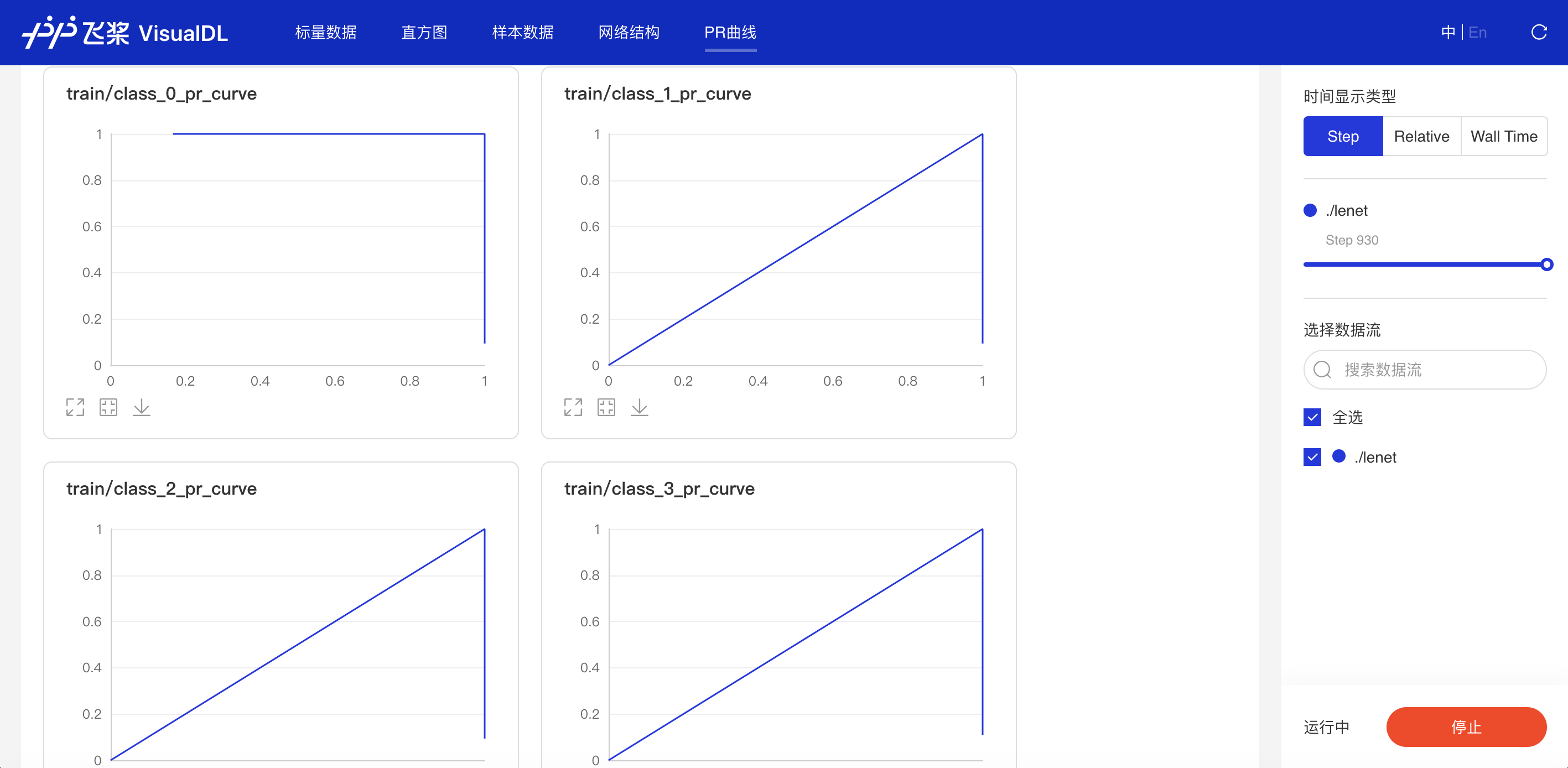### 功能操作说明¶

• 支持数据卡片「最大化」，「还原」、「下载」PR曲线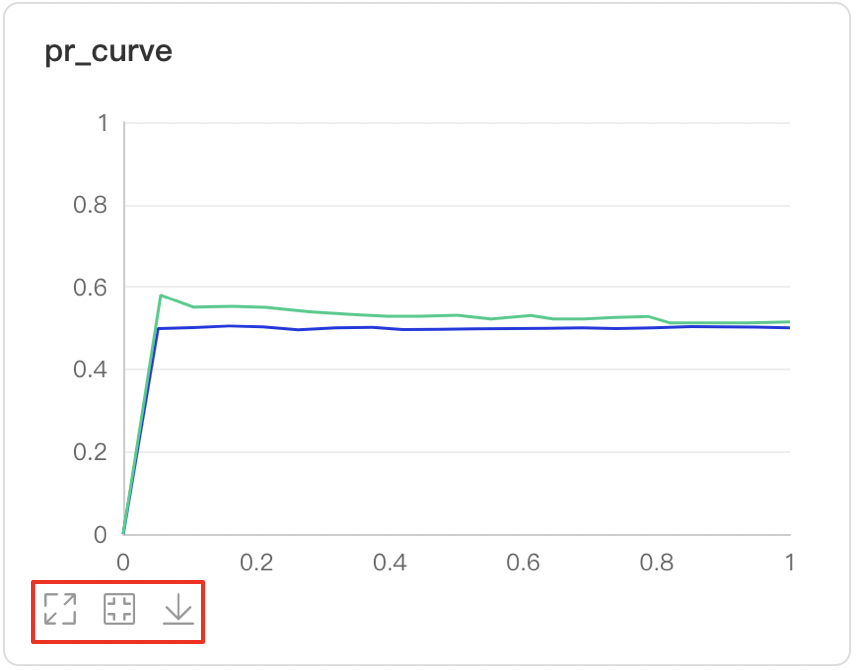• 数据点Hover展示详细信息：阈值对应的TP、TN、FP、FN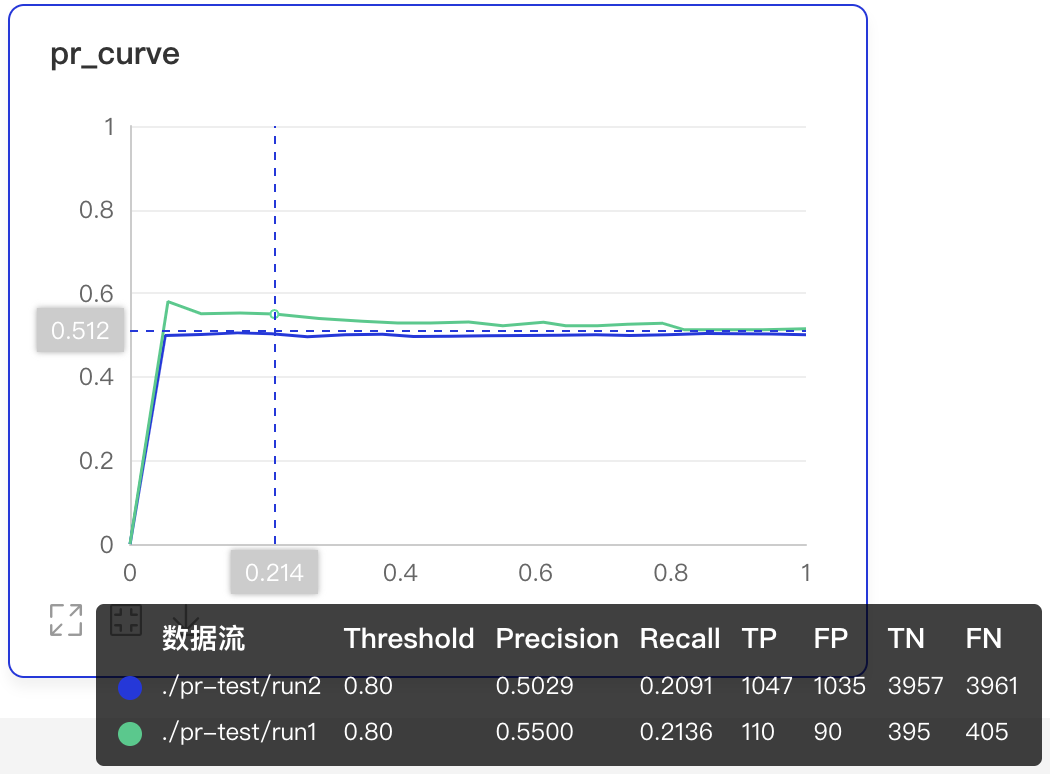• 可搜索卡片标签，展示目标图表• 可搜索打点数据标签，展示特定数据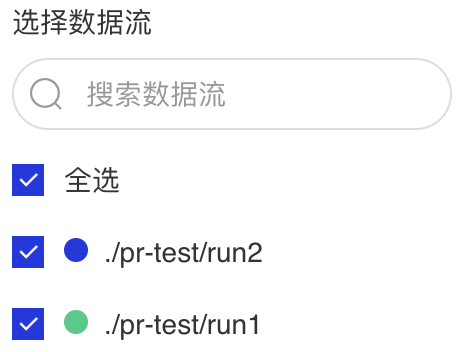• 支持查看不同训练步数下的PR曲线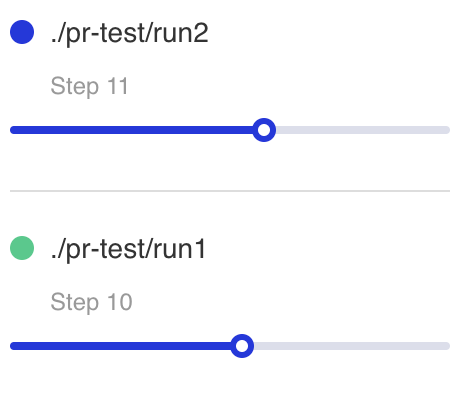• X轴-时间显示类型有三种衡量尺度

• Step：迭代次数

• Walltime：训练绝对时间

• Relative：训练时长## High Dimensional -- 数据降维组件¶

### 介绍¶

High Dimensional 组件将高维数据进行降维展示，用于深入分析高维数据间的关系。目前支持以下两种降维算法：

• PCA : Principle Component Analysis 主成分分析

• t-SNE : t-distributed stochastic neighbor embedding t-分布式随机领域嵌入

### 记录接口¶

High Dimensional 组件的记录接口如下：

```add_embeddings(tag, labels, hot_vectors, walltime=None)
```

tag string 记录指标的标志，如`default`，不能含有`%`
labels numpy.array 或 list 一维数组表示的标签，每个元素是一个string类型的字符串
hot_vectors numpy.array or list 与labels一一对应，每个元素可以看作是某个标签的特征
walltime int 记录数据的时间戳，默认为当前时间戳

### Demo¶

```from visualdl import LogWriter

if __name__ == '__main__':
hot_vectors = [
[1.3561076367500755, 1.3116267195134017, 1.6785401875616097],
[1.1039614644440658, 1.8891609992484688, 1.32030488587171],
[1.9924524852447711, 1.9358920727142739, 1.2124401279391606],
[1.4129542689796446, 1.7372166387197474, 1.7317806077076527],
[1.3913371800587777, 1.4684674577930312, 1.5214136352476377]]

labels = ["label_1", "label_2", "label_3", "label_4", "label_5"]
# 初始化一个记录器
with LogWriter(logdir="./log/high_dimensional_test/train") as writer:
# 将一组labels和对应的hot_vectors传入记录器进行记录
```visualdl --logdir ./log --port 8080